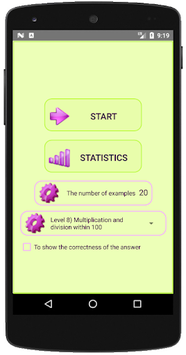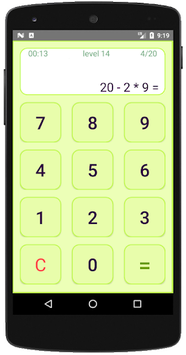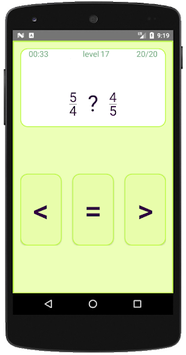# Mental arithmetic. Math workout APK

Training mental arithmetic. Math tests for children from 6 years

### APK Details

 Category Education Last Updated 2018-09-17 Latest Version 10.3 Size 2.74 MB Installations 10000+ Android version 4.0 and up Content Rating Everyone

### Screenshots## Mental arithmetic. Math workout APK Description

The app is designed to teach mental arithmetic.
The app is designed for pupils, students and pupils over 6 years.
The order of levels and their complexity is made up of experienced teachers.
19 levels of complexity.

- Examples are generated in the program, not written initially
- Correctness of answers is checked automatically
- The time of the exercise is calculated automatically
- Possibility to choose the number of examples in the exercise
- The sequence of topics in the school curriculum
- Statistics

Topics:
1. Addition and subtraction within 10
2. Addition and subtraction within 20
3. Chains of examples within a 10
4. Addition and subtraction of two-digit and one-digit
5. Addition and subtraction of numbers, one of which is round
6. Addition and subtraction within 100
7. Multiplication and division by one number
8. Multiplication and division within 100
9. Addition and subtraction within 1000 (round numbers)
10. Chains on addition and subtraction within 100
11. Multiplication and division within 1000 (round numbers)
12. Chains on multiplication and division within 100
13. Mixed chains within 100
14. Chains with brackets
15. Negative number
16. Chains with negative numbers
17. Comparison of fractions
18. Addition and subtraction of fractions
19. Multiplication and division of fractions